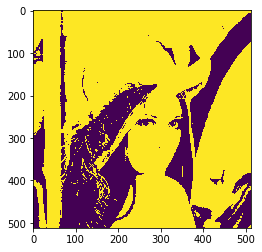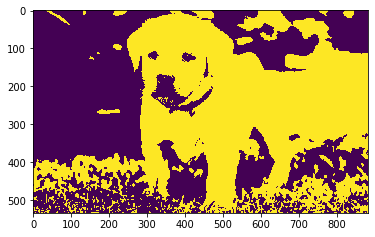Related Articles

# Mahotas – Euler number of Image

• Last Updated : 07 Aug, 2021

In this article, we will see how we can get the euler number of the image in mahotas. The Euler number is a measure of the topology of an image. It is defined as the total number of objects in the image minus the number of holes in those objects. You can use either 4- or 8-connected neighborhoods.

In this tutorial, we will use “lena” image, below is the command to load it.

`mahotas.demos.load('lena')`

Below is the lena imageIn order to do this we will use mahotas.euler method

Syntax : mahotas.euler(img)

Argument : It takes image object as argument

Return : It returns integer

Note: Input image should be filtered or should be loaded as grey

In order to filter the image we will take the image object which is numpy.ndarray and filter it with the help of indexing, below is the command to do this

`image = image[:, :, 0]`

Below is the implementation

## Python3

 `# importing required libraries``import` `mahotas``import` `mahotas.demos``from` `pylab ``import` `gray, imshow, show``import` `numpy as np``  ` `# loading image``img ``=` `mahotas.demos.load(``'lena'``)`  `  ` `# filtering image``img ``=` `img.``max``(``2``)` `# otsu method``T_otsu ``=` `mahotas.otsu(img)  ``  ` `# image values should be greater than otsu value``img ``=` `img > T_otsu``  ` `print``(``"Image threshold using Otsu Method"``)` `# creating a labeled image``marker, n_nucleus ``=` `mahotas.label(img)``  ` `# showing image``imshow(img)``show()`  `# euler number of image of image``euler ``=` `mahotas.euler(img)` `print``(``"Euler Number of Image : "` `+` `str``(euler))`

Output :

`Image threshold using Otsu Method``Euler Number of Image : 54.25`

Another example

## Python3

 `# importing required libraries``import` `mahotas``import` `numpy as np``from` `pylab ``import` `gray, imshow, show``import` `os`` ` `# loading image``img ``=` `mahotas.imread(``'dog_image.png'``)`  `# filtering image``img ``=` `img[:, :, ``0``]``  ` `# otsu method``T_otsu ``=` `mahotas.otsu(img)  ``  ` `# image values should be greater than otsu value``img ``=` `img > T_otsu``  ` `print``(``"Image threshold using Otsu Method"``)``  ` `# showing image``imshow(img)``show()`  `# euler number of image of image``euler ``=` `mahotas.euler(img)` `print``(``"Euler Number of Image : "` `+` `str``(euler))`

Output :

`Image threshold using Otsu Method ``Euler Number of Image : 76.75`

Attention geek! Strengthen your foundations with the Python Programming Foundation Course and learn the basics.

To begin with, your interview preparations Enhance your Data Structures concepts with the Python DS Course. And to begin with your Machine Learning Journey, join the Machine Learning – Basic Level Course

My Personal Notes arrow_drop_up